# 使用3D变换¶

## 简介¶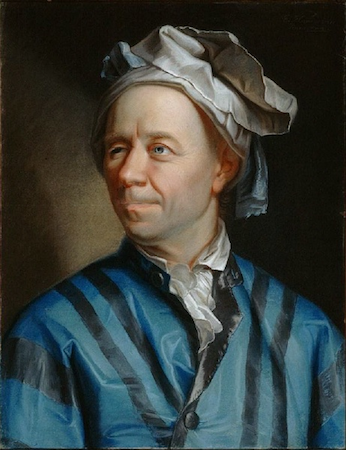## 欧拉角问题¶

### 轴顺序¶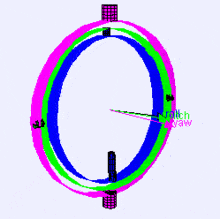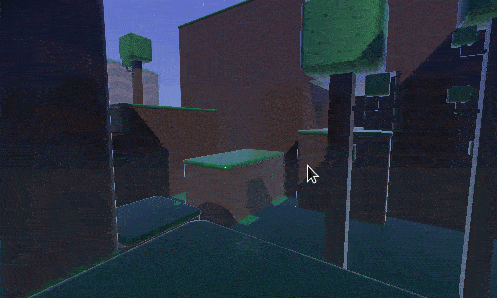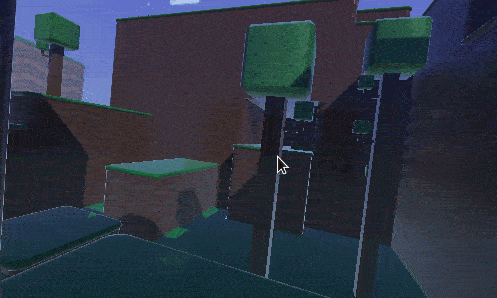### 插值¶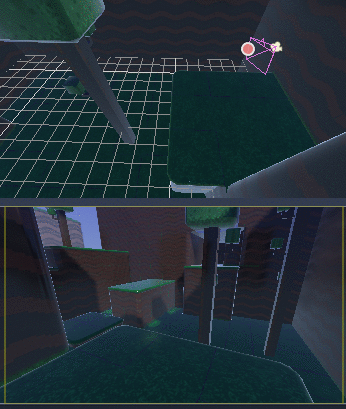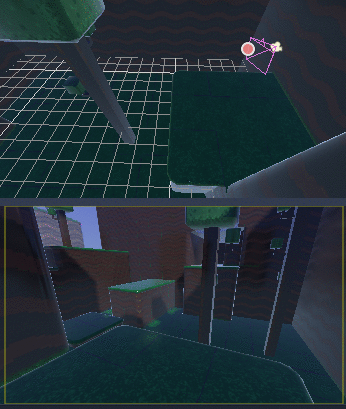• 旋转不会线性映射到方向，因此插入它们并不总是会形成最短路径(即从``270``到``0``的度数与从``270``开始到``360``的度数不同，即使角度是相同的)。
• 万向节锁正在发挥作用(第一个和最后一个旋转的轴对齐，因此失去了一定的自由度)。 请参阅 维基百科关于Gimbal Lock的页面 以了解这个问题的详细解释。

## 变换的介绍¶

Godot对方向使用 class_Transform 数据类型。 每一个 class_Spatial 节点都包含一个 `transform` 属性，如果该父类是一个空间派生类型，则该属性相对依赖于父类变换。

```var basis = Basis()
# Contains the following default values:
basis.x = Vector3(1, 0, 0) # Vector pointing along the X axis
basis.y = Vector3(0, 1, 0) # Vector pointing along the Y axis
basis.z = Vector3(0, 0, 1) # Vector pointing along the Z axis
```
```// Due to technical limitations on structs in C# the default
// constructor will contain zero values for all fields.
var defaultBasis = new Basis();
GD.Print(defaultBasis); // prints: ((0, 0, 0), (0, 0, 0), (0, 0, 0))

// Instead we can use the Identity property.
var identityBasis = Basis.Identity;
GD.Print(identityBasis.x); // prints: (1, 0, 0)
GD.Print(identityBasis.y); // prints: (0, 1, 0)
GD.Print(identityBasis.z); // prints: (0, 0, 1)

// The Identity basis is equivalent to:
var basis = new Basis(Vector3.Right, Vector3.Up, Vector3.Back);
GD.Print(basis); // prints: ((1, 0, 0), (0, 1, 0), (0, 0, 1))
```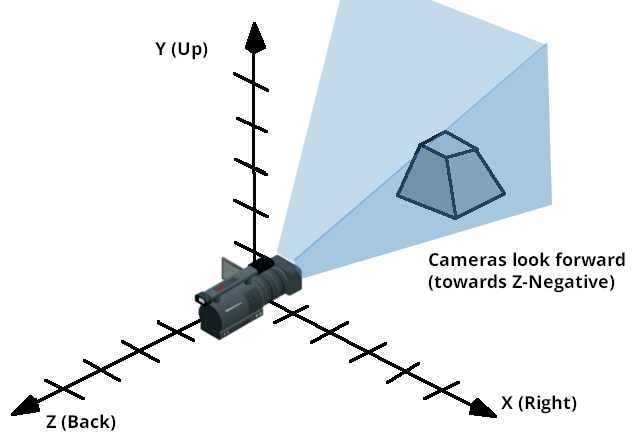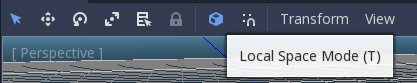Gizmo的箭头显示基准的“X”，“Y”和“Z”轴(分别为红色，绿色和蓝色)，而Gizmo的中心位于对象的原点。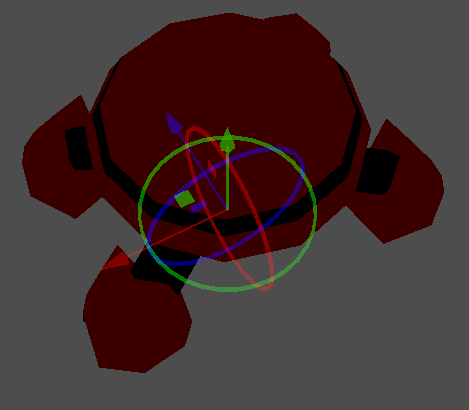### 操作变换¶

```# Rotate the transform about the X axis
transform.basis = Basis(Vector3(1, 0, 0), PI) * transform.basis
# shortened
transform.basis = transform.basis.rotated(Vector3(1, 0, 0), PI)
```
```// rotate the transform about the X axis
transform.basis = new Basis(Vector3.Right, Mathf.Pi) * transform.basis;
// shortened
transform.basis = transform.basis.Rotated(Vector3.Right, Mathf.Pi);
```

Spatial中的一种方法简化了这一点:

```# Rotate the transform in X axis
rotate(Vector3(1, 0, 0), PI)
# shortened
rotate_x(PI)
```
```// Rotate the transform about the X axis
Rotate(Vector3.Right, Mathf.Pi);
// shortened
RotateX(Mathf.Pi);
```

```# Rotate locally
rotate_object_local(Vector3(1, 0, 0), PI)
```
```// Rotate locally
RotateObjectLocal(Vector3.Right, Mathf.Pi);
```

### 精度误差¶

```transform = transform.orthonormalized()
```
```transform = transform.Orthonormalized();
```

```transform = transform.orthonormalized()
transform = transform.scaled(scale)
```
```transform = transform.Orthonormalized();
transform = transform.Scaled(scale);
```

### 获取信息¶

```bullet.transform = transform
bullet.speed = transform.basis.z * BULLET_SPEED
```
```bullet.Transform = transform;
bullet.LinearVelocity = transform.basis.z * BulletSpeed;
```

```# Get the direction vector from player to enemy
var direction = enemy.transform.origin - player.transform.origin
if direction.dot(enemy.transform.basis.z) > 0:
enemy.im_watching_you(player)
```
```// Get the direction vector from player to enemy
Vector3 direction = enemy.Transform.origin - player.Transform.origin;
if (direction.Dot(enemy.Transform.basis.z) > 0)
{
enemy.ImWatchingYou(player);
}
```

```# Remember that +X is right
if Input.is_action_pressed("strafe_left"):
translate_object_local(-transform.basis.x)
```
```// Remember that +X is right
if (Input.IsActionPressed("strafe_left"))
{
TranslateObjectLocal(-Transform.basis.x);
}
```

```# Keep in mind Y is up-axis
if Input.is_action_just_pressed("jump"):
velocity.y = JUMP_SPEED

velocity = move_and_slide(velocity)
```
```// Keep in mind Y is up-axis
if (Input.IsActionJustPressed("jump"))
velocity.y = JumpSpeed;

velocity = MoveAndSlide(velocity);
```

### 设置信息¶

```# accumulators
var rot_x = 0
var rot_y = 0

func _input(event):
if event is InputEventMouseMotion and event.button_mask & 1:
# modify accumulated mouse rotation
rot_x += event.relative.x * LOOKAROUND_SPEED
rot_y += event.relative.y * LOOKAROUND_SPEED
transform.basis = Basis() # reset rotation
rotate_object_local(Vector3(0, 1, 0), rot_x) # first rotate in Y
rotate_object_local(Vector3(1, 0, 0), rot_y) # then rotate in X
```
```// accumulators
private float _rotationX = 0f;
private float _rotationY = 0f;

public override void _Input(InputEvent @event)
{
if (@event is InputEventMouseMotion mouseMotion)
{
// modify accumulated mouse rotation
_rotationX += mouseMotion.Relative.x * LookAroundSpeed;
_rotationY += mouseMotion.Relative.y * LookAroundSpeed;

// reset rotation
Transform transform = Transform;
transform.basis = Basis.Identity;
Transform = transform;

RotateObjectLocal(Vector3.Up, _rotationX); // first rotate about Y
RotateObjectLocal(Vector3.Right, _rotationY); // then rotate about X
}
}
```

### 四元数插值¶

```# Convert basis to quaternion, keep in mind scale is lost
var a = Quat(transform.basis)
var b = Quat(transform2.basis)
# Interpolate using spherical-linear interpolation (SLERP).
var c = a.slerp(b,0.5) # find halfway point between a and b
# Apply back
transform.basis = Basis(c)
```
```// Convert basis to quaternion, keep in mind scale is lost
var a = transform.basis.Quat();
var b = transform2.basis.Quat();
// Interpolate using spherical-linear interpolation (SLERP).
var c = a.Slerp(b, 0.5f); // find halfway point between a and b
// Apply back
transform.basis = new Basis(c);
```

class_Quat 类型的引用有更多关于数据类型的信息(它也可以做变换累加，变换点等等，虽然这个用法较少)。 如果多次对四元数进行插值或应用运算，请记住它们需要最终归一化，否则它们可能会产生数值精度错误。